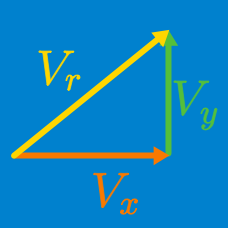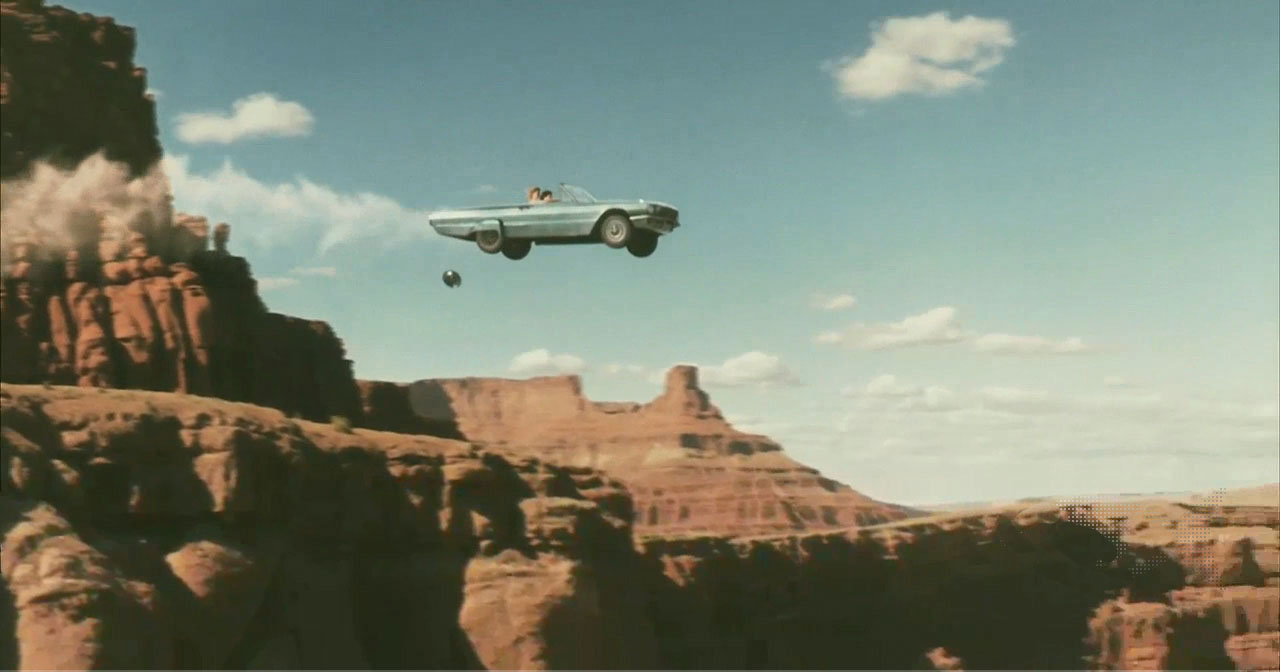Classical Mechanics

# Problem solving with vector kinematics - beginners

A lady goes on a hike for $90 \text{ minutes}.$ Her average speed is $8 \text{ km/h},$ and she hikes in a direction that makes a $60^\circ$ angle with the eastward and a $30^\circ$ angle with the northward. Express her displacement using two direction components.

Assume that $\sqrt{3}$ is approximately $1.7.$

The motion of a creature on a two dimensional plane can be described by the following equations for velocity in $x \text{ and } y$ directions: \begin{aligned} v_x(t)&=6t \\ v_y(t)&=- 2t + 7. \\ \end{aligned} Find the square of the magnitude of its velocity vector at $t = 2.$

Immediately after a ship sets out to sail $150 \text{ km}$ north, an unexpected storm blows the ship to a point $140 \text{ km}$ east of its starting point. Approximately how far must it now sail to reach its original destination?

A car drives at $40.0 \text{ km/h}$ due west for $60 \text{ minutes},$ then due north at $20.0 \text{ km/h}$ for $90 \text{ minutes}.$ Find the magnitude of the car's total displacement.A car drives straight off the edge of a cliff that is 80 m high. At the scene of the accident you note that the point of impact is 160m from the base of the cliff. How fast was the car traveling in m/s when it went over the cliff?

Consider $g = 10 \text{ m/s}^2$

×